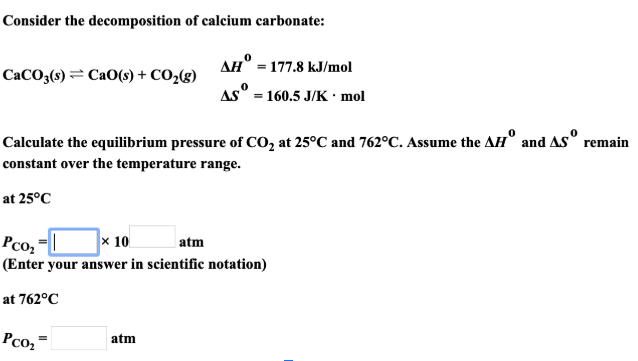# Consider the decomposition of calcium carbonate: CaCO3(s) ⇌ CaO(s) + CO2(g) ΔH° = 177.8 kJ/mol ΔS° = 160.5 J/K.mol Calculate the equilibrium pressure of CO2 at 25°C and 762°C. Assume the ΔH° and ΔS° remain constant over the temperature range. at 25°C at 762°C